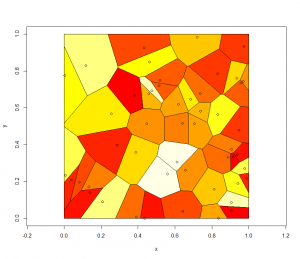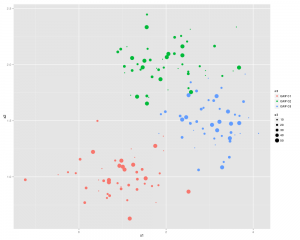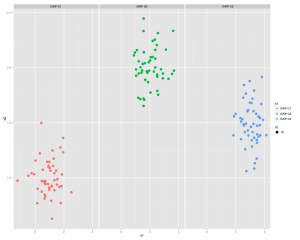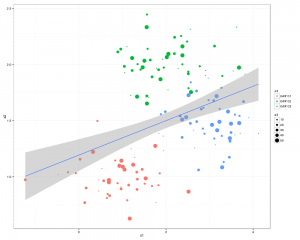# QGIS not so happy about hi DPI

A default install of QGIS doesn’t work too well with a HiDPI display.

However setting an environment variable seem to sort all that out.

```export QT_AUTO_SCREEN_SCALE_FACTOR=true
```

# Voronoi in R (from deldir manual)```install.packages("deldir")

install.packages("deldir")
library(deldir)
set.seed(42)
x <- runif(50)
y <- runif(50)
z <- deldir(x,y,rw=c(0,1,0,1))
w <- tile.list(z)
plot(w)
ccc <- heat.colors(20) # Or topo.colors(20), or terrain.colors(20)
# or cm.colors(20), or rainbow(20).
```

# ggplot2/qplot basics

Install and load the ggplot2 and Cairo libraries

```install.packages(c("ggplot2","Cairo")
library(c(ggplot2,Cairo))
```

set up some data (or use some real data)

```x1<-rnorm(150,mean = rep(1:3, each =50),sd = 0.7)
x2<-rnorm(150,mean = rep(c(1,2,1.5), each = 50),sd = 0.2)
x3<-rnorm(150,mean = rep(c(20,30,3),each = 50)), sd = 0.5)
n3<-rep(c("GRP 01","GRP 02","GRP 03"),each=50)
```

Here is the command to generate the PNG file, with anti-aliasing:

```CairoPNG(filename = "Plot1.png", antialias="subpixel", width = 1000, height=800, units = "px")
{
qplot(x1,x2, ,color = n3, size = x3)
}
dev.off()
```or you can split the 3 sections up using:

` qplot(x1,x2, color = n3, facets = .~n3)`# ...and now something similar using GGPLOT2

First thing we need to do is create a dataframe from the four identical length vectors.

```df <- data.frame(x1,x2,x3,n3)
colnames(df) <- c("x1","x2","x3","n3")```

Some Charting:

```g1 <- ggplot(df,aes(x1,x2))
p <- g1 + geom_point(aes(colour=n3), size =3.5) +
geom_smooth(method = "lm") +
theme_bw()
print(p)
```

..and a slightly better looking version:

```g1 <- ggplot(df,aes(x1,x2))
p  <- g1 + geom_point(aes(colour=n3, size =x3)) +
geom_smooth(method = "lm") +
theme_bw()
print(p)
```There you go all good stuff.
Other things to check out: facet_wrap
Some more pretty graphics

# treemap in R

```library(RODBC)
library(lattice)
library(treemap)

ch<-odbcConnect("mike_db",uid="mike")
c<-sqlQuery(ch, paste("select"
,"ward,year(end_Dttm) as [year]"
,",sum(datediff(mi,start_Dttm,end_Dttm)/1440.0) as LOS"
,"from [wardstays_examples]"
,"GROUP BY ward ,year(end_Dttm)"
))
str(c)

treemap (c
,index=c("year","ward") # the different levels
,vSize = "LOS" # the value on which to scale the squares
)

```

# GIS in R cran

```library(maptools)
library(Cairo)